# Texas Go Math Kindergarten Lesson 8.6 Answer Key Count and Order to 20

Refer to our Texas Go Math Kindergarten Answer Key Pdf to score good marks in the exams. Test yourself by practicing the problems from Texas Go Math Kindergarten Lesson 8.6 Answer Key Count and Order to 20.

## Texas Go Math Kindergarten Lesson 8.6 Answer Key Count and Order to 20

Explore

DIRECTIONS: Place counters in the ten frames as you count from 1 to 20. Now start with 20. Remove counters from the ten frames as you count backward from 20.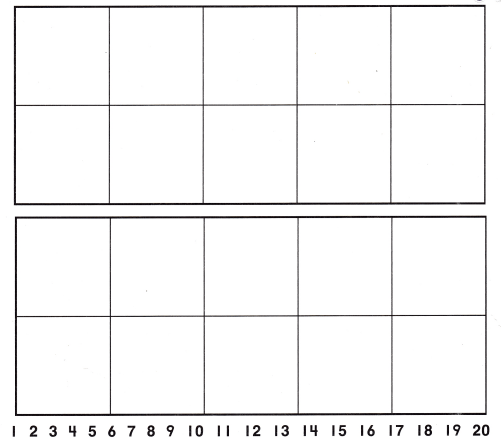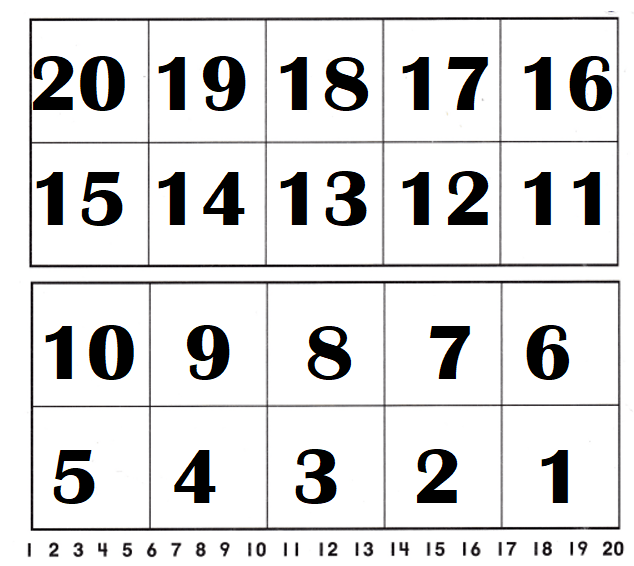Explanation:
Number of counters = 10 + 10 = 20 or Twenty.

Share and Show

DIRECTIONS: 1. Place counters in the ten frame to model each number as shown. Write the number as you count forward. 2. Now start with 10. Remove counters from the ten frame as you count backward. Trace and write the number as you count backward.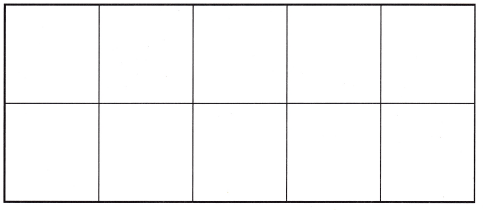Question 1.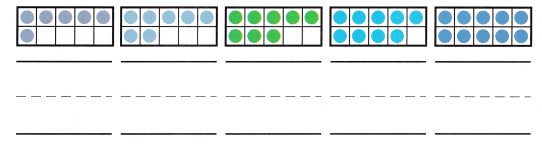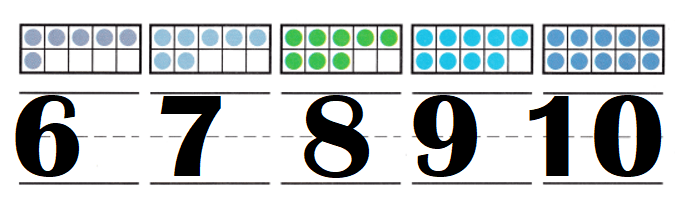Explanation:
Number of counters in the frame = 6, 7,8,9,10.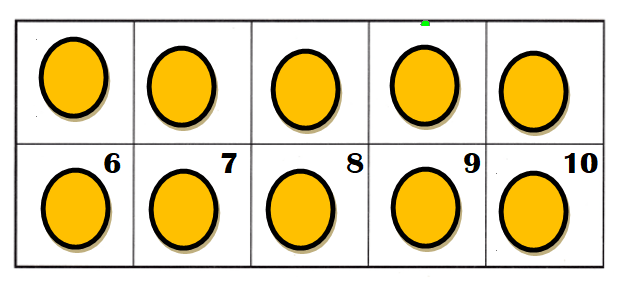Question 2.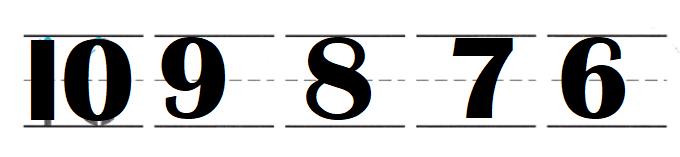Explanation:
Number from backward from 10 = 10, 9, 8, 7, 6, 5, 4, 3, 2, 1.

DIRECTIONS: 3. Place counters in the ten frame to model each number as shown. 4. Write the number as you count forward. Now start with 20. Remove counters from the ten frame as you count backward.

Question 3.Explanation:
Number of counters = 10 or Ten.

Question 4.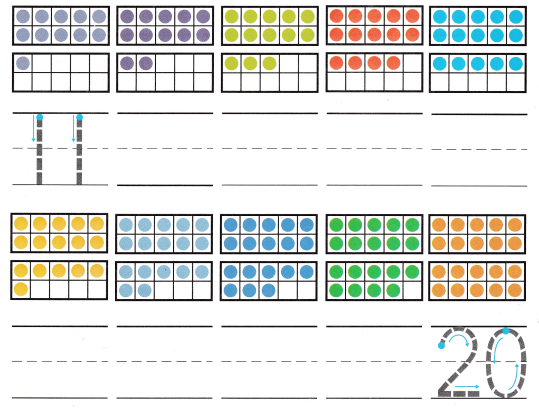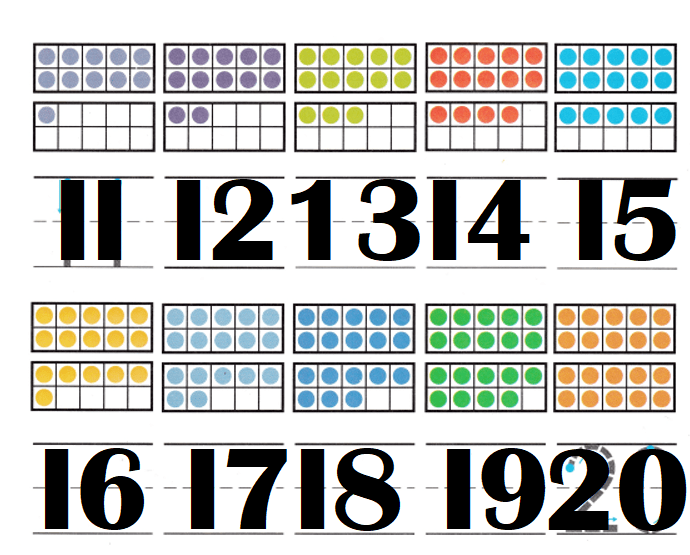Explanation:
Number from 20 to backward = 20, 19, 18, 17, 16, 15, 14, 13, 12, 11.

HOME ACTIVITY • Have your child count a set of objects up to 20 and tell how many.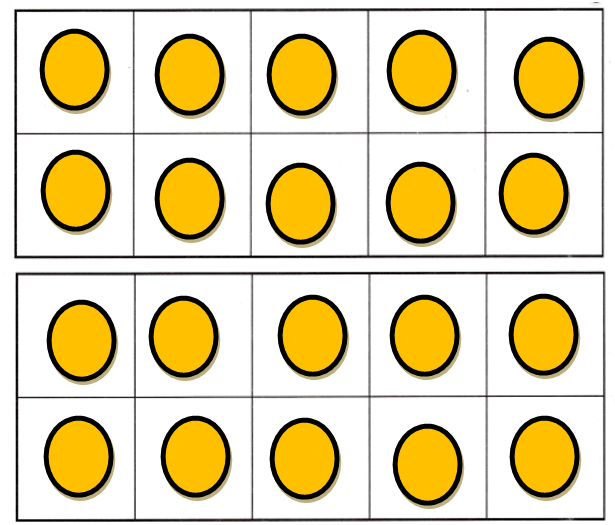Explanation:
My kid took buttons of count 20.

DIRECTIONS: 5. Write to show the numbers in order. Count forward to 20. 6. Choose the correct answer. What is the missing number?

Problem Solving
Question 5.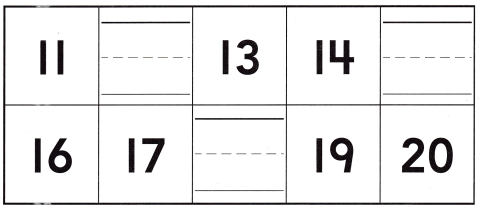Explanation:
Numbers from 11, 12, 13, 14, 15, 16, 17, 18, 19, 20.

Question 6.Explanation:
Numbers from 17 = 17, 18, 19, 20.
Missing number = 19.

### Texas Go Math Kindergarten Lesson 8.6 Homework and Practice Answer Key

DIRECTIONS: 1. Place counters in the ten frames as you count forward from 11 to 20. 2. Now start with 20. Remove counters from the ten frame as you count backward to 11. Trace and write the number as you count backward from 20.

Question 1.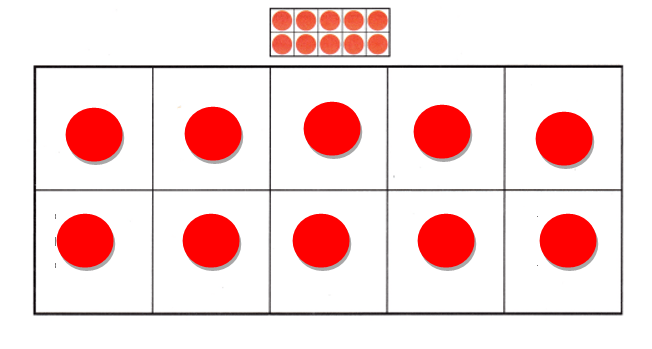Explanation:
Number count from 10 = 11, 12, 13, 14,15, 16, 17,18,19, 20.

Question 2.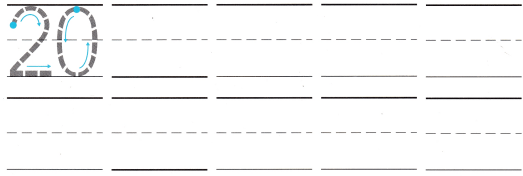Explanation:
Numbers backward from 20 = 20, 19, 18, 17, 16, 15, 14, 13, 12, 11, 10.

DIRECTIONS: Choose the correct answer. 3-5. What is the missing number?

Lesson Check
Question 3.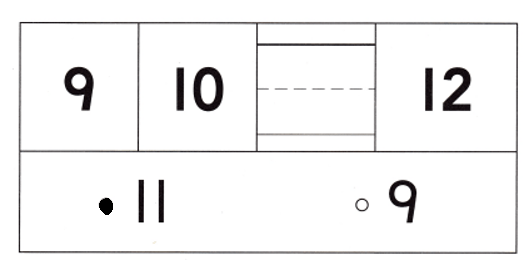Explanation:
Number from 9 = 9, 10, 11, 12.
Missing number = 11.

Question 4.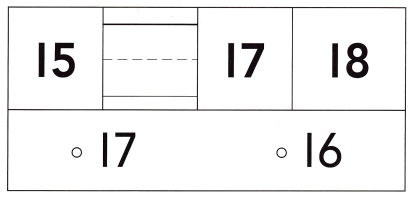Explanation:
Number from 15 = 15, 16, 17, 18.
Missing number = 16.

Question 5.